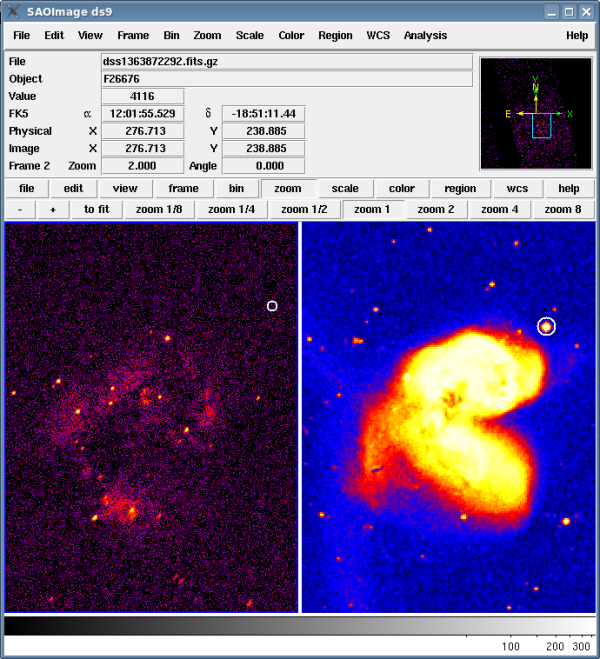# Computing the Intensity Upper Limit for an Unresolved Source## Overview

Last Update: 27 Jan 2010 - updated for CIAO 4.2: use N004 version of data products from the Archive; screen output updated

Synopsis:

The tool aprates, computes values and bounds for source intensity quantities (net counts, source rate, photon flux, energy flux) using counts and exposure data obtained in source and background apertures, as shown in the Compute Net Counts, Rate, or Flux for Point Sources thread.

This thread uses aprates to determine how x-ray bright a source could be at the given location in the observation. The intensity upper limit is calculated in flux units in this thread.

aprates uses Bayesian statistics to compute the background-marginalized, posterior probability distribution for source intensity, assuming non-informative prior distributions for background and source intensity (for details on the algorithm, see the Background Marginalized X-ray Source Intensity memo). The posterior distribution can be used to determine intensity value and confidence bounds or intensity upper limit.

you want to calculate the intensity upper limit for a source within a specified aperture.

## Get Started

Sample ObsID used: 315 (ACIS-S, NGC 4038/39)

## Selecting the Apertures

Figure 1 shows the x-ray data (left) and an optical image from the Digital Sky Survey (right). The optical image was retrieved from SAO-DSS via the ds9 "Analysis → Image Servers" menu. The star circled in white - 2MASS J12014790-1851156 - is clearly visible in the optical but not in x-ray.

### Figure 1: Apertures overlaid on x-ray and optical data### Figure 1: Apertures overlaid on x-ray and optical data

The left frame contains the Chandra x-ray data and the right frame contains an optical image from the DSS. The location (ra,dec)=(12:01:47.9, -18:51:15.6) is circled in both frames.

The location (ra,dec)=(12:01:47.9, -18:51:15.6) will be used as the center of the apertures in this thread. A circle with radius 5" is used as the source region and an annulus with inner radius of 5" and outer radius of 10" is used for the background:

```circle(12:01:47.9,-18:51:15.6,5")

annulus(12:01:47.9,-18:51:15.6,5",15")
```

## Counts in source and background apertures

The number of counts in the source (n) and background (m) apertures is found by running dmlist on the event file with the source and background regions. The energy range of the events is restricted to 0.5-7.0 keV:

```unix% dmlist 'acisf00315N004_evt2.fits[energy=500:7000][(ra,dec)=circle(12:01:47.9,-18:51:15.6,5")]' counts
14

unix% dmlist 'acisf00315N004_evt2.fits[energy=500:7000][(ra,dec)=annulus(12:01:47.9,-18:51:15.6,5",15")]' counts
130
```

There are 14 source counts (n=14) and 130 background counts (m=130).

## Areas of source and background apertures

The area of the source (A_s) and background (A_b) apertures is computed:

```Source:   5^2 * pi = 78.5

Background: (15^2 - 5^2) * pi = 628.3
```

The source area is 78.5 square arcsec (A_s=78.5) and the background area is 628.3 square arcsec (A_b=628.3).

## Exposure time of the observation

By setting the exposure times in the source (T_s) and background (T_b) apertures, the net source rate and errors will be computed.

For this example, the exposure time is the same for the source and background since they come from the same file:

```unix% dmkeypar acisf00315N004_evt2.fits LIVETIME echo+
72241.5027948
```

The exposure time is 72241.50 s.

## Run aprates to find the limit

For this calculation, it is assumed that 99% of the PSF is contained within the source region (alpha=.99) and 1% is in the background region (beta=.01). The following parameters are all set to "1" as we are not calculating flux: E_s, eng_s, flux_s, E_b, eng_b, and flux_b.

The aprates parameters are set with the values determined above and the tool is run. For details on how the tool does the calculations, refer to the aprates help file.

```unix% punlearn aprates
unix% pset aprates n=14 m=130
unix% pset aprates A_s=78.5 A_b=628.3
unix% pset aprates alpha=0.99 beta=0.01
unix% pset aprates T_s=72241.50 T_b=72241.50
unix% pset aprates E_s=1 eng_s=1 flux_s=1 E_b=1 eng_b=1 flux_b=1
unix% pset aprates outfile=ulimit.par

unix% aprates mode=h
# aprates (CIAO 4.2): WARNING: Large number of counts, just using Gaussian pdf
```

The warning indicates that Gaussian statistics were used instead of Poisson statistics. This is controlled by the max_counts parameter: when n+m > max_counts, Gaussian statistics are used.

The output file is in parameter file format, which makes it possible to query the output values with the tool pget:

```unix% pget ulimit.par src_rate src_rate_err_lo src_rate_err_up
0
INDEF
5.60511e-05
```

At the 68% confidence level, the upper limit on the x-ray count rate is 5.60511e-05 counts/s.

The full output file, ulimit.par, is included at the end of the thread.

```

src_cnts,r,h,0.0,,,
src_cnts_err_lo,r,h,INDEF,,,
src_cnts_err_up,r,h,4.04921,,,
src_cnts_conf,r,h,0.682686,,,
src_cnts_status,i,h,0,,,
src_cnts_signif,r,h,0,,,
src_cnts_mode,r,h,0,,,
src_rate,r,h,0.0,,,
src_rate_err_lo,r,h,INDEF,,,
src_rate_err_up,r,h,5.60511e-05,,,
src_rate_conf,r,h,0.682686,,,
src_rate_status,i,h,0,,,
src_rate_signif,r,h,0,,,
src_rate_mode,r,h,0,,,
photflux_aper,r,h,0.0,,,
photflux_aper_err_lo,r,h,INDEF,,,
photflux_aper_err_up,r,h,4.04921,,,
photflux_aper_conf,r,h,0.682686,,,
photflux_aper_status,i,h,0,,,
photflux_aper_signif,r,h,0,,,
photflux_aper_mode,r,h,0,,,
flux_aper,r,h,0.0,,,
flux_aper_err_lo,r,h,INDEF,,,
flux_aper_err_up,r,h,4.04921,,,
flux_aper_conf,r,h,0.682686,,,
flux_aper_status,i,h,0,,,
flux_aper_signif,r,h,0,,,
flux_aper_mode,r,h,0,,,
eflux_aper,r,h,0.0,,,
eflux_aper_err_lo,r,h,INDEF,,,
eflux_aper_err_up,r,h,4.04921,,,
eflux_aper_conf,r,h,0.682686,,,
eflux_aper_status,i,h,0,,,
eflux_aper_signif,r,h,0,,,
eflux_aper_mode,r,h,0,,,
# ---- Inputs below ----
_n,i,h,14,,,
_A_s,r,h,78.5,,,
_alpha,r,h,0.99,,,
_T_s,r,h,72244.7,,,
_E_s,r,h,1,,,
_eng_s,r,h,1,,,
_flux_s,r,h,1,,,
_m,i,h,130,,,
_A_b,r,h,628.3,,,
_beta,r,h,0.01,,,
_T_b,r,h,72241.5,,,
_E_b,r,h,1,,,
_eng_b,r,h,1,,,
_flux_b,r,h,1,,,
```

## History

 24 Jul 2009 New for CIAO 4.1: title updated to "Computing the Intensity Upper Limit for an Unresolved Source" 27 Jan 2010 updated for CIAO 4.2: use N004 version of data products from the Archive; screen output updated